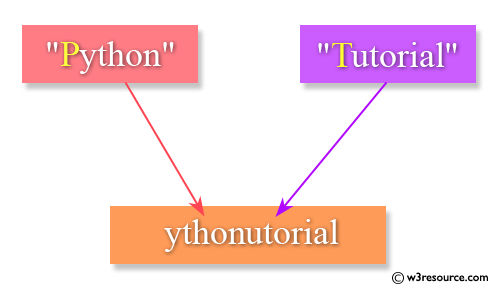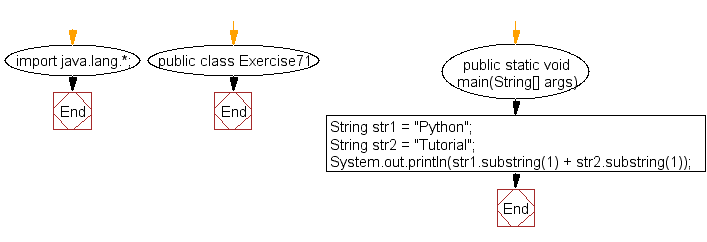﻿ Java: Add 2 strings except by removing the first character# Java Exercises: Create the concatenation of the two strings except removing the first character of each string

## Java Basic: Exercise-71 with Solution

Write a Java program to create the concatenation of the two strings except removing the first character of each string. The length of the strings must be 1 and above.

Pictorial Presentation:Sample Solution:

Java Code:

``````import java.lang.*;
public class Exercise71 {
public static void main(String[] args)
{
String str1 = "Python";
String str2 = "Tutorial";

System.out.println(str1.substring(1) + str2.substring(1));
}
}
```
```

Sample Output:

```ythonutorial
```

Flowchart:Java Code Editor:

What is the difficulty level of this exercise?

Test your Programming skills with w3resource's quiz.

﻿

## Java: Tips of the Day

countOccurrences

Counts the occurrences of a value in an array.

Use Arrays.stream().filter().count() to count total number of values that equals the specified value.

```public static long countOccurrences(int[] numbers, int value) {
return Arrays.stream(numbers)
.filter(number -> number == value)
.count();
}
```

Ref: https://bit.ly/3kCAgLb